# SAT Math Multiple Choice Question 95: Answer and Explanation

### Test Information

Question: 95

5. If -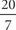< -3z + 6 < -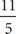, what is the greatest possible integer value of 9z - 18 ?

• A. 6
• B. 7
• C. 8
• D. 9

CWhen solving inequalities, the natural impulse is to isolate the variable. In this case, though, look at what the question is asking. The question doesn't want you to find just the the value of z but rather the value of 9z - 18. To get from the value of -3z + 6 given in the inequality to this new value, the original inequality must be multiplied by -3. Just multiply the entire inequality by this value, making sure to flip the inequality signs when multiplying by a negative number. The equation becomes -3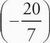> -3(-3z + 6) > -3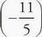or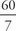> 9z - 18 >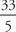. The question asks for the greatest possible integer value, so focus on the high end of the given values. The value at that end,, equals 8.57, so the greatest integer less than that is 8. The answer is (C).